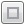1
Double-click the column name to which you want to apply the formula, select Formula from the Column Properties menu, and then click Edit Formula.
 – When you type, a small text editor window appears, which enables you to edit the formula. You can click the Maximize editor buttonif you need more room. Long formulas open in the maximized editor by default.
 – When you select a column or function, that item is added to the selected blue box.
 – You can also drag a column from the Columns list into the selected box.
 2 Add expressions, functions, and terms. Then they appear in the highlighted blue box. The following sections in this chapter provide detailed instructions on how to add constants, elements, operators, and functions.
Figure 6.2 Building a Formula
See Use Basic Formula Editor Features, for an example of how to use the Formula Editor.
Notes:
 • In a formula, when you reference a column using value labels, place your cursor over the value label to see the actual data value.
 • Whitespace in a formula is automatically removed by JMP.
 • You can also use the Formula Editor to define a custom format. For example, you might want to convert a number from inches to centimeters using the calculation Char( :height * 2.54 ) || " cm". Realize that the underlying data, which you see when double-clicking a data table cell, would still be the value of :height.
 • To move the cursor from one field to another in a formula, select the field and press the left arrow key.
`:Y - Col Mean( If( :Status == "nonsmoker", :Y, . ), :Cycle )`
In a new Y2 column, create this formula:
`If( :Status == "nonsmoker",`
`	:Y,`
`	.`
`)`
`:Y - Col Mean( :Name( :Y2, :Cycle )`

Help created on 7/12/2018